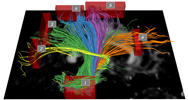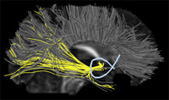DTI-Query 1.1 CINCH 1.0 BETA

### .pdb Format

This document describes the binary format of .pdb files (pathway database files) for use with both CINCH and DTI-Query. Note that all values are stored in little-endian format, regardless of the endianness of the machine used to compute the pathways. Integers are 4 bytes, characters are 1 byte each, doubles and longs are 8 bytes.

```[ pdb header size, in bytes ] - integer

-- PDB HEADER FOLLOWS --

[ 4x4 transformation matrix which is used to transform from voxel space to scanner space (or some other space) ] - 16 doubles, stored in row-major order (same as VTK).

[ number of pathway statistics ] - integer

for each statistic:
[ currently unused ] - integer
[ is this statistic stored per point, or just as an aggregate per path? ] - integer (0 = false, 1 = true)
[ currently unused ] - integer
[ name of the statistic ] - char
[ currently unused ] - char
[ unique ID - an integer that uniquely identifies this statistic, across files ] - integer

[ number of algorithms ] - integer

for each algorithm:
[ algorithm name ] - char
[ comments about the algorithm ] - char
[ unique ID ] - an integer that uniquely identifies this algorithm, across files ] - integer

[ version number ] - integer (DTIQuery 1.1 = 1, this field did not exist prior to DTIQuery 1.1)

-- PDB HEADER ENDS --

[ number of pathways ] - integer

for each pathway:
[ header size ] - integer

-- PATHWAY HEADER FOLLOWS --

[ number of points ] - integer
[ algorithm ID ] - integer
[ seed point index ] - integer, currently unused by DTI-Query

for each statistic:
[ precomputed statistical value ] - double

-- PATHWAY HEADER ENDS --

for each point:
[ position of the point - in 0-indexed voxel space! ] - 3 doubles
for each statistic:
IF the statistic is also computed per point (see statistics header, second field):
for each point:
[ statistical value for this point ] - double

for each pathway:
[ byte offset of pathway header ] - long

-- END OF FILE --
```

Questions? Problems? E-mail us.